# Medians 2:1

Median to side b (tb) in triangle ABC is 12 cm long.
a. What is the distance of the center of gravity T from the vertex B?
b, Find the distance between T and the side b.

Correct result:

x =  8 cm
y =  4 cm

#### Solution:

$t=12 \ \text{cm} \ \\ x=\dfrac{ 2 }{ 3 } \cdot \ t=\dfrac{ 2 }{ 3 } \cdot \ 12=8 \ \text{cm}$
$y=t-x=12-8=4 \ \text{cm}$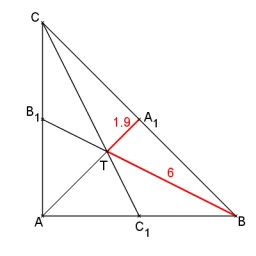Our examples were largely sent or created by pupils and students themselves. Therefore, we would be pleased if you could send us any errors you found, spelling mistakes, or rephasing the example. Thank you!

Leave us a comment of this math problem and its solution (i.e. if it is still somewhat unclear...):Be the first to comment!Tips to related online calculators
Need help calculate sum, simplify or multiply fractions? Try our fraction calculator.
Do you want to convert length units?

#### You need to know the following knowledge to solve this word math problem:

We encourage you to watch this tutorial video on this math problem:

## Next similar math problems:

• Divide in ratioLine segment AB 12 cm long divide in a ratio of 5: 3. How long are the individual parts?
• Tourist routeHow long is the tourist route when tourists crossed four-sevenths of the way on foot, crossed the bus twice less than on foot and passed the last 14 kilometers by boat.
• What time is it?What time is it, when there is 4 times less time left until midnight than the time that has elapsed since noon? What time is it, when one-fifth of the hours that have passed since midnight is equal to one-third of the hours that are missing by 12 o'clock?
• Half of halvesHalf of the square we cut off, then half of the rest, etc. Five cuts we made in this way. What part of the content of the original square is the content of the cut part?
• Pizza palaceJosh is at Enzo's pizza palace. He can sit at a table with 5 of his friends or at a different table with seven of his friends. The same size pizza is shared equally among the people at each table. At which table should Josh sit to get more pizza? (write a
• Ratio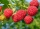Alena collected 7.8 kg of blueberries, 2.6 kg of blackberries, and 3.9 kg of cranberries. Express the ratio in the smallest natural numbers in this order.
• SummandsWe want to split the number 110 into three summands so that the first and the second summand are in the ratio 4: 5, and the third with the first are in ratio 7: 3. Calculate the smallest of the summands.
• The tourist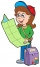The tourist walked a quarter of the way on the first day, a third of the rest on the second day, and 20 km on the last day. How many km did he walked in three days?
• A farmer's heritage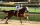A farmer died leaving his 17 horses to his 3 sons. When his sons opened up the Will it read: My eldest son should get 1/2 (half) of total horses; My middle son should be given 1/3rd (one-third) of the total horses; My youngest son should be given 1/9th (
• Double ratio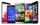The mobile phone was twice gradually discounted in the ratio of 3: 2 1 half: 5 quarters. How much did it originally cost if the price was CZK 4,200 after a double discount?
• Five combers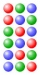Five combers harvest 12 rows of strawberries in 4 hours. How many rows of strawberries will two combers harvest in 10 hours?
• The dough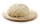The dough contains water, flour, and sugar. Water and flour in a ratio of 2: 3, flour, and sugar in a ratio of 2: 1. Determine the ratio of all three components of the dough.
• Age of familyThe age of father and son is in the ratio 10: 3. The age of the father and daughter is in ratio 5: 2. How old are a father and a son if the daughter is 20?
• Evaluate expressionCalculate the value of the expression z/3 - 2 z/9 + 1/6, for z = 2
• Brass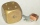Brass is an alloy of copper and zinc in a ratio of 3: 2. How many grams does a component that required 270 g of copper weigh?
• The number 72The number 72 increase by 25%. By how much % will you have to reduce the number you created to get the number 72 again?Adam has half the money in his right pocket than in his left pocket. If he transferred 40 crowns from the left pocket to the right, he would have the same in both pockets. Calculate how many crowns does Adam have in his left pocket more than in his right?